[Introduction]  [Signal arithmetic]  [Signals and noise]   [Smoothing]   [Differentiation]  [Peak Sharpening]  [Harmonic analysis]   [Fourier convolution]  [Fourier deconvolution]  [Fourier filter]  [Wavelets]   [Peak area measurement]  [Linear Least Squares]  [Multicomponent Spectroscopy]  [Iterative Curve Fitting]  [Hyperlinear quantitative absorption spectrophotometry] [Appendix and Case Studies]  [Peak Finding and Measurement]  [iPeak]   [iSignal]  [Peak Fitters]   [iFilter]  [iPower]  [List of downloadable software]  [Interactive tools]

###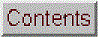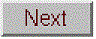Appendix S. Measuring signal-to-noise ratio in complex signals. (Added October, 2016)

In the section of Signals and Noise, I said: "The quality of a signal is often expressed as the signal-to-noise (S/N) ratio, which is the ratio of the true signal amplitude ... to the standard deviation of the noise." That's a simple enough statement, but automating the measurement of signal and the noise in real signals is not always straightforward. Sometimes it's difficult to separate or distinguish between the signal and the noise, because it depends not only on the numerical nature of the data, but also on the objectives of the measurement.

For a simple DC (direct current) signal, for example measuring a fluctuating voltage, the signal is just the average voltage value and the noise is its standard deviation. This is easily calculated in a spreadsheet or in Matlab/Octave:

>> signal=mean(NoisyVoltage);
>> noise=std(NoisyVoltage);
>> SignalToNoiseRatio=signal/noise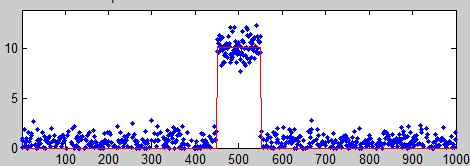But usually things are more complicated. For example, if the signal is a rectangular pulse (as in the figure on the left) with constant random noise, then the simple formulation above will not give accurate results. If the signal is stable enough that you can get two successive signal recordings m1 and m2 that are identical except for the noise, then you can simply subtract the signal out: the standard deviation of the noise is then given by sqrt((std(m1-m2)2)/2), where std is the standard deviation function (because random noise adds quadratically). But not every signal source is stable and repeatable enough for that to work perfectly. Alternatively, you can try to measure the average signal just over the top of the pulse and the noise only over the baseline interval before and/or after the pulse. That's not so hard to do by hand, but it's harder to automate with a computer, especially if the position or width of the pulse changes. It's basically the same for smooth peak shapes like the commonly-encountered Gaussian peak (as in the figure on the right)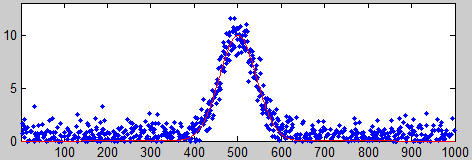. You can estimate the height of the peak by smoothing it and then taking the maximum of the smoothed peak as the signal: max(fastsmooth(y,10,3)), but the accuracy would degrade if you choose too high or two low a smooth width. And clearly all this depends on having a well-defined baseline in the data where there is only noise. It doesn't work if the noise varies with the amplitude of the peak.

In many cases, curve fitting can be helpful. For example, you could use peak fitting or a peak detector to locate multiple peaks and measure their peak heights and their S/N ratios on a peak-to-peak basis, by computing the noise as the standard deviation of difference between the raw data and the best-fit line over the top part of the peak. That's how iSignal measures S/N ratios of peaks. Also, iSignal has baseline correction capabilities that allow the peak to be measured relative to the nearby baseline.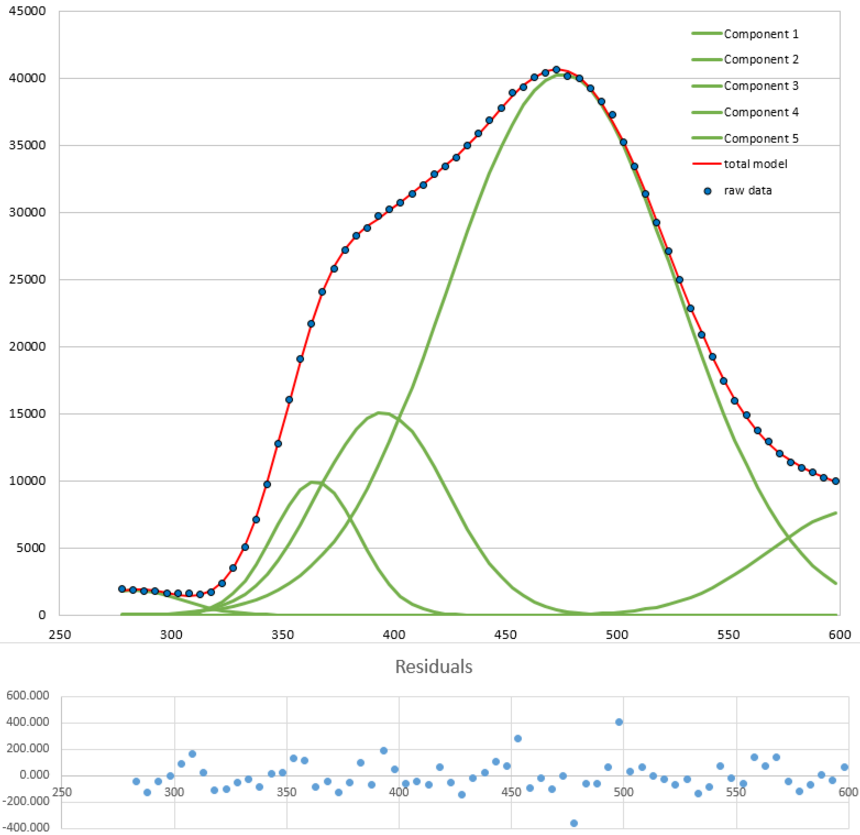Curve fitting also works for complex signals of indeterminate shape that can be approximated by a high-order polynomial or as the sum of a number of basic functions such as Gaussians, as in the example shown on the left. In this example, five Gaussians are used to fit the data to the point where the residuals are random and unstructured. The residuals (shown in blue below the graph) are then just the noise remaining in the signal, whose standard deviation is easily computed using the built-in standard deviation function in a spreadsheet ("STDEV") or in Matlab/Octave ("std"). In this example, the standard deviation of the residuals is 111 and the maximum signal is 40748, so the percent relative standard deviation of the noise is 0.27% and the S/N ratio is 367. (The positions, heights, and widths of the Gaussian components, usually the main results of the curve fitting, are not used in this case; curve fitting is used only to obtain a measure the noise via the residuals). The advantage of this approach over simply subtracting two successive measurements of the signal is that it adjusts for slight changes in the signal from measurement to measurement; the only assumption is that the signal is a smooth, low-frequency waveform that can be fit with a polynomial or a collection of basic peak shapes and that the noise is random and mostly high-frequency compared the the signal.  But don't use too high a polynomial order' otherwise you are just "fitting the noise".

With periodic signal waveforms the situation is a bit more complicated. As an example, consider the audio recording of the spoken phrase "Testing, one, two, three" (click to download in .mat format or in WAV format) that was used previously. The Matlab/Octave script PeriodicSignalSNR.m loads the audio file into a variable "waveform", then computes the average amplitude of the waveform (the "envelope") by smoothing the absolute value of the waveform:

envelope = fastsmooth(abs(waveform), SmoothWidth, SmoothType);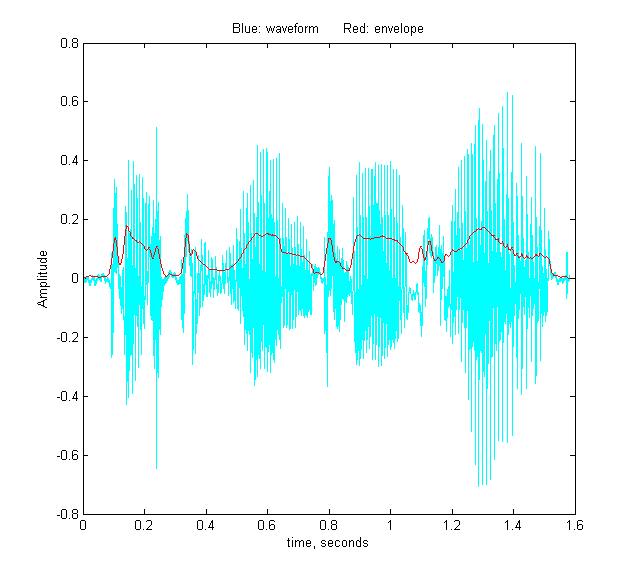The result is plotted on the left, where the waveform is in blue and the envelope is in red. The signal is easy to measure as the maximum or perhaps the average of the waveform, but the noise is not so evident. The human voice is not reproducible enough to get a second identical recording to subtract out the signal as above. Still, there will be often be gaps in the sound, during which the background noise will be dominant. In an audio (voice or music) recording, there will typically be such gaps at the beginning, then the recording process has already started but the sound has not yet begun, and possibly at other short periods when there are pauses in the sound. The idea is that, by monitoring the envelope of the sound and noting when it falls below some adjustable threshold value, we can automatically record the noise that occurs in those gaps, whenever they may occur in a recording. In PeriodicSignalSNR.m, this operation is done in lines 26-32, and the threshold is set in line 12. The threshold value has to be optimized for each recording. When the threshold value is set to 0.015 in the "Testing, one, two, three" recording, the resulting noise segments are located and are marked in red in the plot on the right.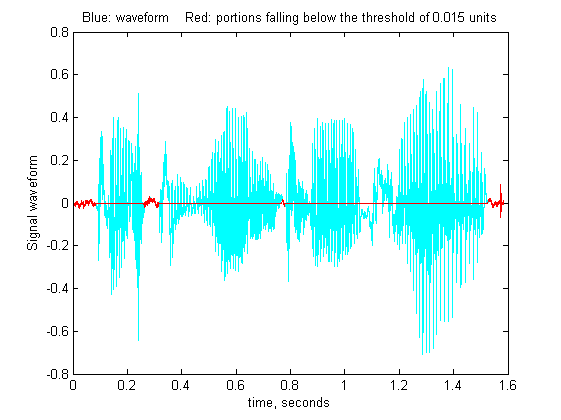The program determines the average noise level in this recording simply by computing the standard deviation of those segments (line 46), then computes and prints out the peak-to-peak S/N ratio and the RMS (root mean square) S/N ratio.

PeakToPeak_SignalToNoiseRatio = 143.7296

RMS_SignalToNoiseRatio = 12.7966

The frequency distribution of the noise is also determined (lines 60-61) and shown in the figure on the left,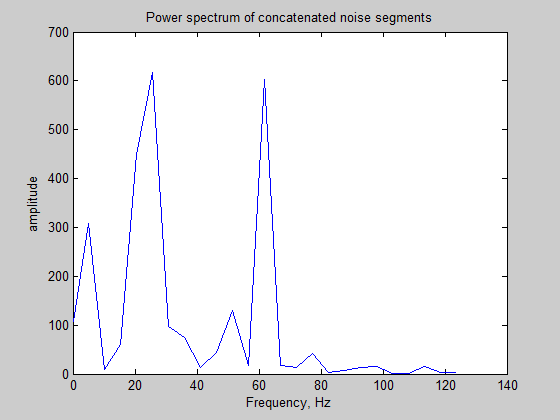using the PlotFrequencySpectrum function, or you could have used iSignal in the frequency spectrum mode (Shift-S). The spectrum of the noise shows a strong component very near 60 Hz, which is almost certainly due to power line pickup (the recording was made in the USA, where AC power is 60Hz); this suggests that better shielding and grounding of the electronics might help to clean up future recordings. The lack of strong components at 100 Hz and above suggests that the vocal sounds have been effectively suppressed at this threshold setting. The script can be applied to other sound recordings in WAV format simply by changing the file name and time axis in lines 8 and 9.

This page is part of "A Pragmatic Introduction to Signal Processing", created and maintained by Prof. Tom O'Haver , Department of Chemistry and Biochemistry, The University of Maryland at College Park. Comments, suggestions and questions should be directed to Prof. O'Haver at toh@umd.edu. Updated July, 2022.